# AP Calculus AB Practice Test 55

### Test Information7 questions21 minutes

Calculator Allowed

1.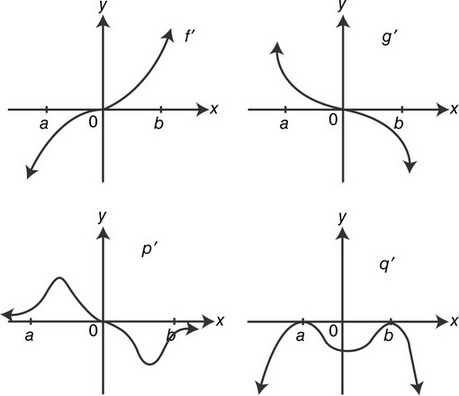What is the volume of the solid obtained by revolving the region enclosed by the graphs of x= y2and x= 9 about the y-axis?

2. At what value(s) of xdo the graphs of y= exand y= x2+ 5xhave parallel tangent lines?

3. Let yrepresent the population in a town. If ydecreases according to the equation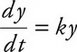, with tmeasured in years, and the population decreases by 25% in 6 years, then k=

4. If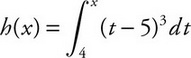on [4, 8], then hhas a local minimum at x=

5. The volume of the solid generated by revolving the region bounded by the graph of y= x3, the line y= 1, and the y-axis about the y-axis is

6.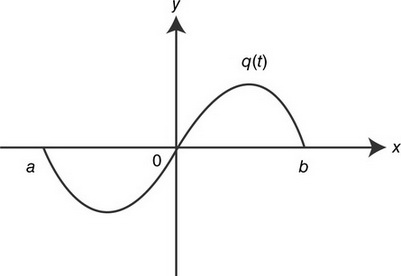If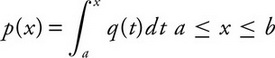and the graph of qis shown above, which of the graphs shown below is a possible graph of p?

7. The equation of the tangent line to the graph of y= sin xfor 0 ≤ xπat the point where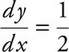is